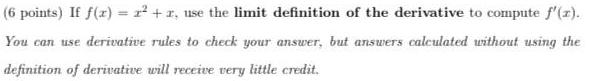Question:

# If f(x) = 2² +1, use the limit definition of the derivative

Last updated: 8/14/2022If f(x) = 2² +1, use the limit definition of the derivative to compute f'(z). You can use derivative rules to check your answer, but answers calculated without using the definition of derivative will receive very little credit.